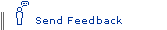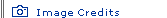The Power of Tiny ThingsAnswer Key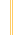Einstein's Big Idea homepage

1. (c) an atomic bomb

It's true. If you could harness its power—that is, turn every one of its atoms into pure energy—the paper clip would yield about 18 kilotons of TNT. That's roughly the size of the bomb that destroyed Hiroshima in 1945. In the Hiroshima explosion, countless atoms of uranium were split apart in a nuclear chain reaction. Each time an atom split, the total mass of the fragments speeding apart was less than that of the original atom. The bomb, in essence, transformed three-hundredths of an ounce of mass into a cataclysmic burst of heat and light.

2. (a) kill an amoeba

That's right. Two hundred million electron volts couldn't even boil a thimbleful of water but would likely kill an amoeba. Splitting a single atom of uranium yields a tiny amount of energy. When we speak of the awesome "power of the atom," we are generally referring to chain reactions involving as many as one septillion atoms—that's 1 followed by 24 zeros!

3. (b) four pounds

Yes. If the solar energy that strikes Earth each second were converted to mass, it would equal nearly four pounds. The sun's energy is generated by nuclear fusion: at the heart of the sun, under tremendous pressure, hydrogen atoms fuse together to form helium. Each new atom of helium, though, has less mass than the four original hydrogen atoms that created it. The "lost" mass radiates off as light that can warm the Earth 93 million miles away.

4. (b) power New York State for about two days

Correct. Of course, on Earth there is no practical way to convert pennies, paper clips, or other objects entirely to energy. It would require temperatures and pressures greater than those at the core of our sun. In theory, though, a dollar's worth of change could satisfy all of New York State's energy needs for about two days. Humankind uses so much energy in a month that it would take about 1,000 pounds worth of change.

5. (c) 29 million years

Yes. If every grain became energy, the lump would yield around 25 billion kilowatt-hours—enough to keep the bulb glowing for about 29 million years. Burning the coal in a conventional power plant, by contrast, produces only enough energy to keep the bulb shining for 67 hours. In an Einsteinian dream world, power generation could be billions of times more efficient than ordinary combustion—and leave behind no ash or smoke!

6. (c) possibly split Earth in half

Yes, and it probably wouldn't even take a McMansion. People in the business of relocating entire houses estimate that a 3,000-square-foot house weighs about 60 tons. Transformed entirely to energy, such a house would yield the equivalent of about 1,170,000 megatons of TNT, which would be roughly 65 million times more powerful than the bomb that fell on Hiroshima. This is far more energy than would be needed to melt half the ice in Antarctica—and possibly enough to split Earth in half.

7. (a) a flea

Correct. It's as if a flea has hitched a ride. Even zooming at roughly 18,000 mph, the Space Shuttle is nowhere near a speed that would significantly change its mass. When subatomic particles travel near the speed of light, though, as they do in the world's most powerful particle accelerators, they can become as much as 40,000 times heavier than they are at rest.

In Short...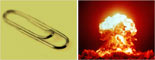The mass of a small paper clip has roughly the energy of the atomic bomb that destroyed Hiroshima.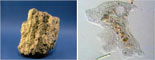When a single atom of uranium is split, less than a proton's worth of mass is converted into a tiny bit of energy—enough to kill an amoeba.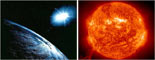All the solar energy that strikes Earth in a second is equivalent to four pounds of mass.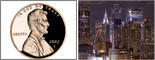A dollar's worth of pennies could power New York State for about two days.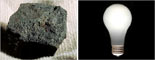A 2.2-pound lump of coal, in theory, could illuminate a light bulb for 29 million years.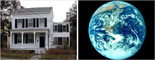A moderate-sized house, converted to energy, could possibly split Earth in half.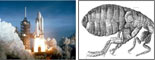When the Space Shuttle travels at its top speed of 18,000 mph, it gains roughly the mass of a flea.

Note on Calculations

While the concept of mass-energy equivalence is mind-boggling, the calculations involved are relatively simple. To figure out the energy inherent in any object, you just need to know its mass, choose the correct units (such as "meters per second" and "Joules"), and do a bit of algebra.

The following Web site walks through a sample problem and even has a handy automatic calculator that allows you to find the energy equivalent for any mass you choose. Have fun with it!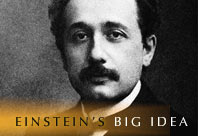Back to the "Einstein's Big Idea" homepage for more articles, interactives, and other features.